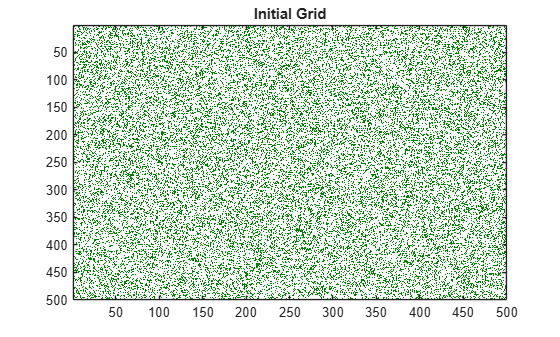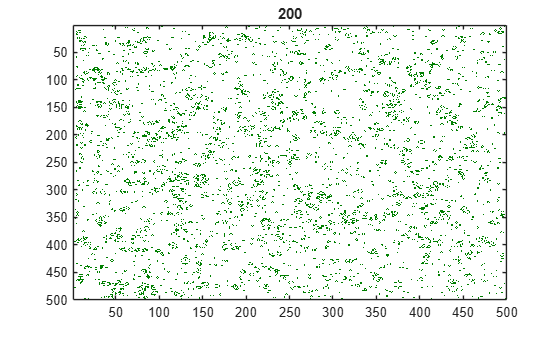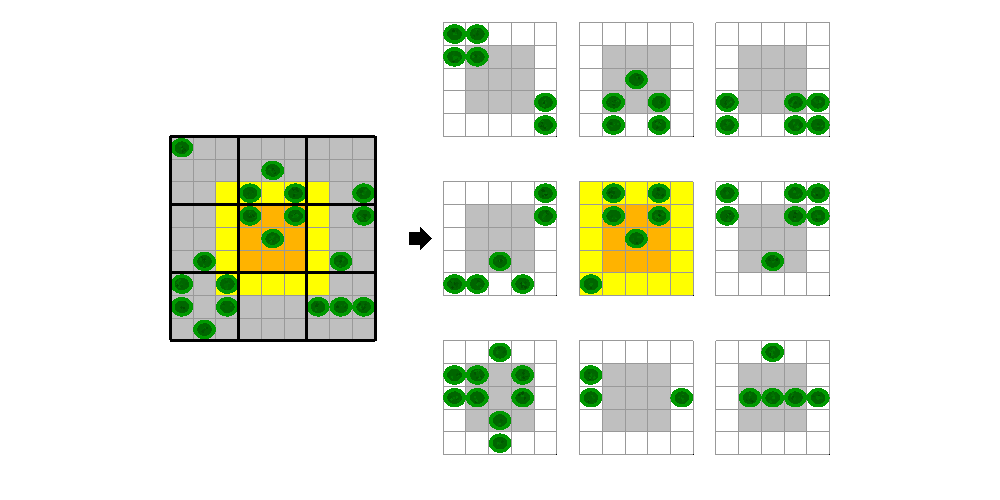# Accessing Advanced CUDA Features Using MEX

This example shows how advanced features of the GPU can be accessed using MEX files. It builds on the example Stencil Operations on a GPU. The previous example uses Conway's "Game of Life" to demonstrate how stencil operations can be performed using MATLAB® code that runs on a GPU. The present example demonstrates how you can further improve the performance of stencil operations using an advanced feature of the GPU: shared memory. You do this by writing your own CUDA code in a MEX file and calling the MEX file from MATLAB. You can find an introduction to the use of the GPU in MEX files in Run MEX Functions Containing CUDA Code.

As defined in the previous example, in a "stencil operation", each element of the output array depends on a small region of the input array. Examples include finite differences, convolution, median filtering, and finite-element methods. If the stencil operation is a key part of your workflow, you can convert it to a hand-written CUDA kernel. This example uses Conway's "Game of Life" as our stencil operation and moves the calculation into a MEX file. The "stencil" in this case is therefore the 3x3 region around each element.

The "Game of Life" follows a few simple rules:

• Cells are arranged in a 2D grid

• At each step, the fate of each cell is determined by the vitality of its eight nearest neighbors

• Any cell with exactly three live neighbors comes to life at the next step

• A live cell with exactly two live neighbors remains alive at the next step

• All other cells (including those with more than three neighbors) die at the next step or remain empty

### Generate a random initial population

Create an initial population of cells on a 2D grid with approximately 25% of the locations alive.

``` gridSize = 500; numGenerations = 200; initialGrid = (rand(gridSize,gridSize) > .75); hold off imagesc(initialGrid); colormap([1 1 1;0 0.5 0]); title('Initial Grid');```### Create a baseline GPU version in MATLAB

To get a performance baseline, start with the initial implementation described in Experiments in MATLAB. Run this implementation on the GPU by making sure the initial population is on the GPU using `gpuArray`. The function `updateGrid` is provided at the end of this example. `updateGrid` counts how many neighbours are alive and decides whether a cell will be alive at the next step.

```currentGrid = gpuArray(initialGrid); % Loop through each generation updating the grid and displaying it for generation = 1:numGenerations currentGrid = updateGrid(currentGrid, gridSize); imagesc(currentGrid); title(num2str(generation)); drawnow; end```Re-run the game and measure how long it takes for each generation. In order to time the entire game using `gputimeit`, a function that calls each generation, `callUpdateGrid`, is provided at the end of this example.

```gpuInitialGrid = gpuArray(initialGrid); % Retain this result to verify the correctness of each version below expectedResult = callUpdateGrid(gpuInitialGrid, gridSize, numGenerations); gpuBuiltinsTime = gputimeit(@() callUpdateGrid(gpuInitialGrid, ... gridSize, numGenerations)); fprintf('Average time on the GPU: %2.3fms per generation \n', ... 1000*gpuBuiltinsTime/numGenerations);```
```Average time on the GPU: 0.521ms per generation ```

### Create a MEX version that uses shared memory

When writing a CUDA kernel version of the stencil operation, you have to split the input data into blocks on which each thread block can operate. Each thread in the block will be reading data that is also needed by other threads in the block. One way to minimize the number of read operations is to copy the required input data into shared memory before processing. This copy must include some neighboring elements to allow correct calculation of the block edges. For the Game of Life, where the stencil is just a 3x3 square of elements, you need a one element boundary. For example, for a 9x9 grid processed using 3x3 blocks, the fifth block would operate on the orange highlighted region, where the yellow elements are the "halo" it must also read.The CUDA code that illustrates this approach is shown in the supporting file `pctdemo_life_cuda_shmem.cu`. To access this supporting file, open this example as a live script. The CUDA device function in this file operates as follows:

1. All threads copy the relevant part of the input grid into shared memory, including the halo.

2. The threads synchronize with one another to ensure shared memory is ready.

3. Threads that fit in the output grid perform the Game of Life calculation.

The host code in this file invokes the CUDA device function once for each generation, using the CUDA runtime API. It uses two different writable buffers for the input and output. At every iteration, the MEX file swaps the input and output pointers so that no copying is required.

In order to call the function from MATLAB, you need a MEX gateway that unwraps the input arrays from MATLAB, builds a workspace on the GPU, and returns the output. The MEX gateway function can be found in the supporting file `pctdemo_life_mex_shmem.cpp`.

To call your own MEX file, you must first compile it using `mexcuda`. Compile `pctdemo_life_cuda_shmem.cu` and `pctdemo_life_mex_shmem.cpp` into a MEX function named `pctdemo_life_mex_shmem`.

`mexcuda -output pctdemo_life_mex_shmem pctdemo_life_cuda_shmem.cu pctdemo_life_mex_shmem.cpp`
```Building with 'NVIDIA CUDA Compiler'. MEX completed successfully. ```
```% Calculate the output value using the MEX file with shared memory. The % initial input value is copied to the GPU inside the MEX file grid = pctdemo_life_mex_shmem(initialGrid, numGenerations); gpuMexTime = gputimeit(@()pctdemo_life_mex_shmem(initialGrid, ... numGenerations)); % Print out the average computation time and check the result is unchanged fprintf('Average time of %2.3fms per generation (%1.1fx faster).\n', ... 1000*gpuMexTime/numGenerations, gpuBuiltinsTime/gpuMexTime);```
```Average time of 0.007ms per generation (72.5x faster). ```
`assert(isequal(grid, expectedResult));`

### Conclusion

This example has illustrated a method of reducing the number of read operations by explicitly copying blocks into shared memory before processing. The performance improvement obtained using this method will depend on the size of the stencil, the size of the overlap region, and the capabilities of your GPU. You can use this approach in conjunction with your MATLAB code to optimize your application.

```fprintf('Using gpuArrays: %2.3fms per generation.\n', ... 1000*gpuBuiltinsTime/numGenerations);```
```Using gpuArrays: 0.521ms per generation. ```
```fprintf(['Using MEX with shared memory: %2.3fms per generation ',... '(%1.1fx faster).\n'], 1000*gpuMexTime/numGenerations, ... gpuBuiltinsTime/gpuMexTime);```
```Using MEX with shared memory: 0.007ms per generation (72.5x faster). ```

### Supporting Functions

This `updateGrid` function updates the 2D grid according to how many neighbours are alive.

```function X = updateGrid(X, N) p = [1 1:N-1]; q = [2:N N]; % Count how many of the eight neighbors are alive neighbors = X(:,p) + X(:,q) + X(p,:) + X(q,:) + ... X(p,p) + X(q,q) + X(p,q) + X(q,p); % A live cell with two live neighbors, or any cell with % three live neighbors, is alive at the next step X = (X & (neighbors == 2)) | (neighbors == 3); end```

The `callUpdateGrid` function calls `updateGrid` for a number of generations.

```function grid=callUpdateGrid(grid, gridSize, N) for gen = 1:N grid = updateGrid(grid, gridSize); end end```